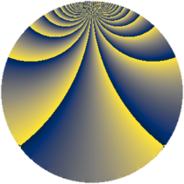Properties

 Label 1859.4.bjLevel $1859$ Weight $4$ Character orbit 1859.bj Rep. character $\chi_{1859}(25,\cdot)$ Character field $\Q(\zeta_{130})$ Dimension $26112$ Sturm bound $728$

Related objects

Defining parameters

 Level: $$N$$ $$=$$ $$1859 = 11 \cdot 13^{2}$$ Weight: $$k$$ $$=$$ $$4$$ Character orbit: $$[\chi]$$ $$=$$ 1859.bj (of order $$130$$ and degree $$48$$) Character conductor: $$\operatorname{cond}(\chi)$$ $$=$$ $$1859$$ Character field: $$\Q(\zeta_{130})$$ Sturm bound: $$728$$

Dimensions

The following table gives the dimensions of various subspaces of $$M_{4}(1859, [\chi])$$.

Total New Old
Modular forms 26304 26304 0
Cusp forms 26112 26112 0
Eisenstein series 192 192 0

Trace form

 $$26112 q - 39 q^{2} - 33 q^{3} - 2201 q^{4} - 39 q^{5} - 39 q^{6} - 39 q^{7} - 39 q^{8} + 4923 q^{9} + O(q^{10})$$ $$26112 q - 39 q^{2} - 33 q^{3} - 2201 q^{4} - 39 q^{5} - 39 q^{6} - 39 q^{7} - 39 q^{8} + 4923 q^{9} - 216 q^{10} - 52 q^{11} - 164 q^{12} + 79 q^{14} - 39 q^{15} + 8551 q^{16} + 71 q^{17} - 39 q^{18} - 39 q^{20} - 104 q^{21} + 1058 q^{22} - 392 q^{23} - 39 q^{24} - 13145 q^{25} + 411 q^{26} + 171 q^{27} - 39 q^{28} - 437 q^{29} - 4087 q^{30} + 2769 q^{31} - 104 q^{32} - 52 q^{33} + 3146 q^{34} - 1801 q^{35} - 18919 q^{36} - 39 q^{37} + 213 q^{38} - 568 q^{39} + 1223 q^{40} - 39 q^{41} - 1653 q^{42} - 3960 q^{43} + 52 q^{44} - 104 q^{45} - 351 q^{46} - 2691 q^{47} + 2387 q^{48} - 28675 q^{49} - 39 q^{50} - 893 q^{51} - 3948 q^{52} + 2239 q^{53} - 104 q^{54} - 7606 q^{55} + 7812 q^{56} - 5733 q^{57} - 39 q^{58} + 4433 q^{59} + 7449 q^{60} - 765 q^{61} + 6733 q^{62} + 7501 q^{63} - 37265 q^{64} - 914 q^{65} - 15787 q^{66} + 26104 q^{67} + 3145 q^{68} - 7116 q^{69} + 5421 q^{71} + 15847 q^{72} - 39 q^{73} - 5787 q^{74} + 12915 q^{75} + 25064 q^{76} + 830 q^{77} - 13140 q^{78} + 2027 q^{79} + 40235 q^{81} + 19097 q^{82} + 12181 q^{83} - 39 q^{84} - 39 q^{85} - 3315 q^{86} - 4012 q^{87} + 5990 q^{88} + 8909 q^{90} - 5354 q^{91} - 10599 q^{92} + 21957 q^{93} + 5299 q^{94} - 7691 q^{95} + 8411 q^{96} - 11037 q^{97} - 104 q^{98} + O(q^{100})$$

Decomposition of $$S_{4}^{\mathrm{new}}(1859, [\chi])$$ into newform subspaces

The newforms in this space have not yet been added to the LMFDB.